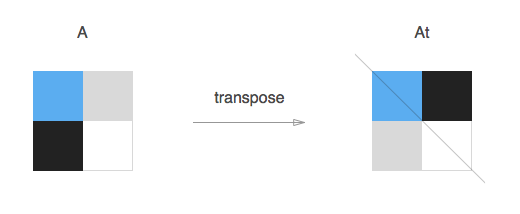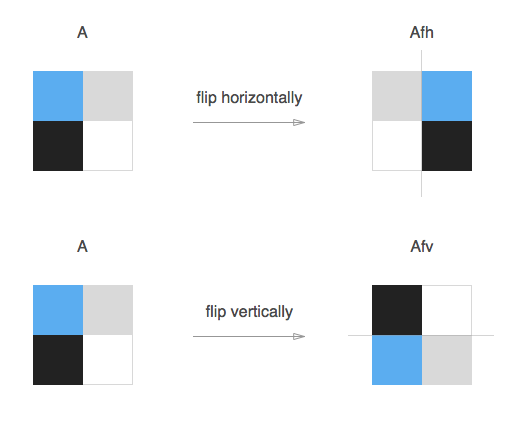# Functional and in-place matrix rotation

## December 23, 2015

In this post I’ll walk through rotating a bi-dimension matrix, in a functional way, by composing transpose and flip functions.
We’ll assume the matrix to be square (n*n).

### Transpose a matrixIn linear algebra, the transpose of a matrix A is another matrix At created by reflecting A over its main diagonal (which runs from top-left to bottom-right) to obtain At. Formally, the i-th row, j-th column element of At is the j-th row, i-th column element of A:

Therefore, a simple function to transpose a Matrix , returning a new transpose of the original, could look something like:

We relied on a deepCopy helper to avoid side effects on the original Matrix. If you were seeking for a constant O(1) space complexity solution, scroll down for the in-place revised version.

### Flip a MatrixThe horizontal flip of a matrix A is another matrix Afh created by reflecting A over its horizontal line of symmetry (which runs from mid-left to mid-right) to obtain Afh.

While the vertical flip of a matrix A is another matrix Afv created by reflecting A over its vertical line of symmetry (which runs from mid-top to mid-bottom) to obtain Afv.

Same idea, slightly different code:

### Rotating

At this point rotating the Matrix, is just a matter of function composition:

• rotate 90 degree clock wise = flipHorizontal(transpose(matrix))
• rotate 90 degree counter clock wise = flipVertical(transpose(matrix))
• rotate 180 degree = flipVertical(flipHorizontal(matrix))

We could then put it all together and have a pretty sweet Matrix rotating function:

### In-place rotation

You are tight in memory and desperately need that O(1) space implementation? Here we go, it will be just a matter of slightly tweaking our transpose and flip functions so that they operate in-place: